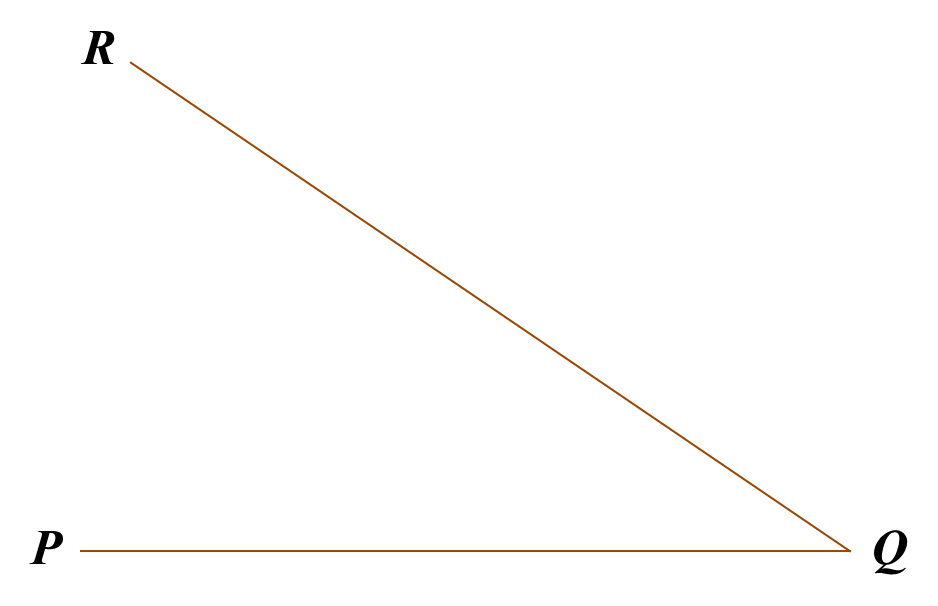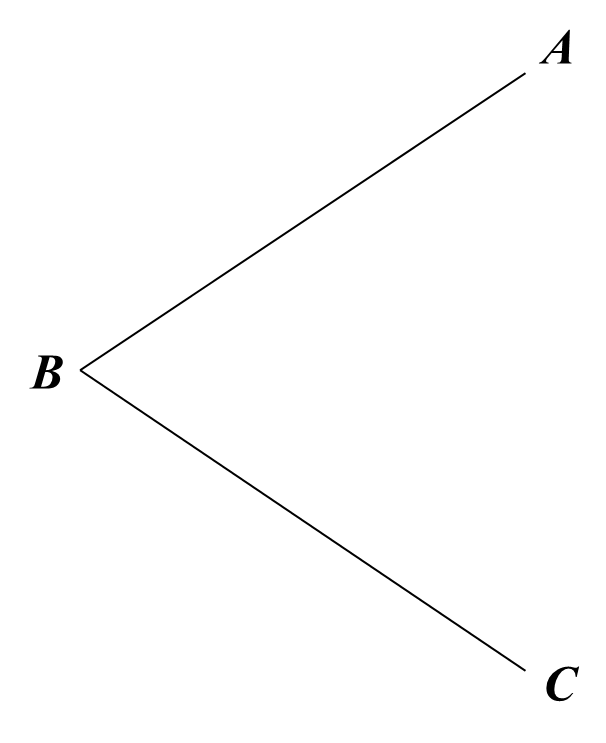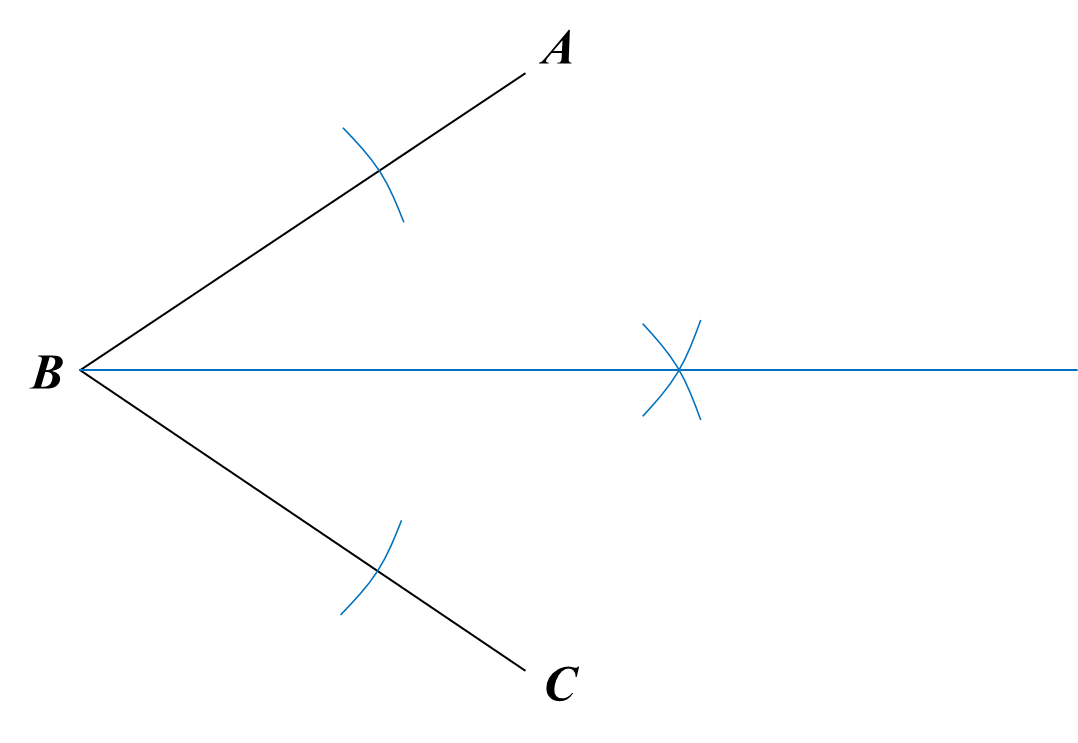# PT3 Mathematics 2016, Question 4

 
      
Question 4 (a):
 
(i) Circle the algebraic expression which has three terms.(ii) Fill in the blank with the correct common factor.(iii) Simplify:
    5a – 1 + a
 
 Solution:
 
(i)(ii)(iii)
 
5a – 1 + a
 
= 5a + a – 1
 = 6a – 1
 
 
 

Question 4 (b):
(i) Measure the acute angle of the ∠PQR using a protractor.(ii) Construct the angle bisector of ABC.(iii) Construct the angle of 120o at A.Solution:
(i)
PQR = 35o

(ii)(iii)Question 4 (c):
 
(i) Diagram 4.1 shows a circle. Arc QR and arc ST are equal in length.Calculate the value of y.
 
 (ii) Diagram 4.2 shows a sector AOB with centre O and the length of arc AB is 11 cm.Calculate the radius, in cm, of sector AOB.
           [   Use π=       22   7     ]          
 
 Solution:
 
(i)
                y+    35    o      +    25    o      =    180    o                y=    180    o          60    o                y=    120    o                     
 
 (ii)
               Arc length AB=    x       360      ×2πr           11=       45       360      ×2×       22    7      ×r           11=       11       14      r           r=       14       11      ×11           r=14 cm